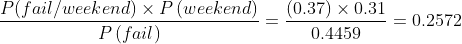Scan QR code or get instant email to install app

Question:

# An educational institute that provides classes for the CFA exam has two batches enrolled for the June 2010 exam. Historically it has been observed that 69% of the candidates enroll in the weekday batch, while the remaining 31% prefer the weekend batch. The historical pass rate for the weekday batch has been 52%, and has been 63% for the weekend batch. Given that a student failed the exam, the probability that she was from the weekend batch is closest to:

A 25.72%.
explanation

1212This question requires us to use Bayes’ Formula for updated probability.
We have to find P (weekend |failure).
We are given the following information:
P (weekday) = 0.69
P (weekend) = 0.31
P (pass| week day) = 0.52
P (pass| weekend) = 0.63
We can infer the following:
P (fail| week day) = 1 − P (pass| weekday) = 1 – 0.52 = 0.48
P (fail| weekend) = 1 – P (pass| weekend) = 1 − 0.63 = 0.37
P (fail)= P(fail| weekday) x P (weekday) +P(fail| weekend) x P(weekend)= (0.48)(0.69) + (0.37)(0.31) = 0.4459
P (weekend| fail)=or 25.72%